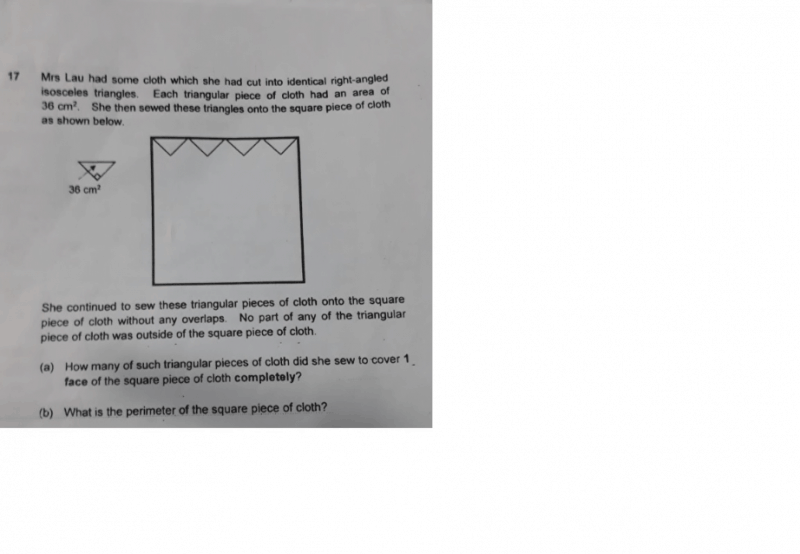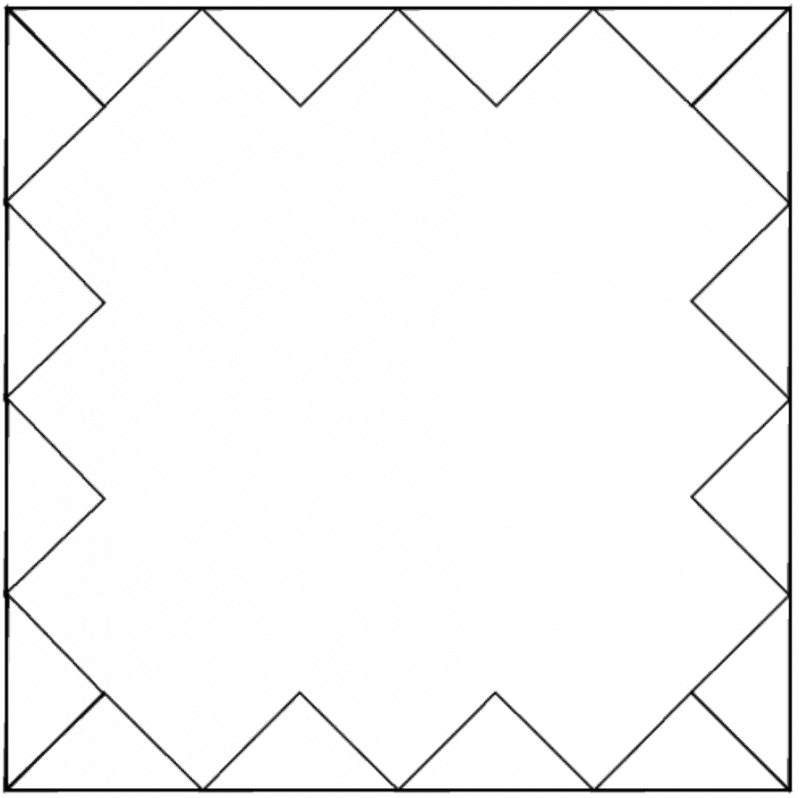# QuestionCan you please help with this question?

1 Answer

# Answera) The important thing to note is that right-angled triangles can be tessellated perfectly onto the square cloth. Are sides are equal and the angles at the sides are both 45°.

It requires some visualization but after sewing 4 triangles onto all four sides, the middle space will be made up of 24 squares. Each square can contain 2 triangles so a total of 48 triangles. I have attached a picture for reference.

So the total number of triangles will be 48 in the middle and the 16 at the corners:
48 + 16 = 64 triangles

b) Total area of all triangles = 64 x 36 cm2
= 2304 cm2

Total area of all triangles = Area of square cloth

Area of square cloth = 2304 cm2

2304 cm2 = 48 cm x 48 cm

Perimeter of square cloth = 48 cm x 4
= 192 cm

0 Replies 0 Likes